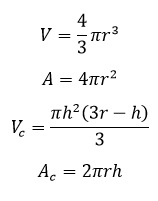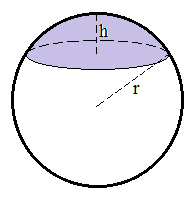# Sphere Calculator

This CalcTown calculator calculates the various parameters related to a sphere

#### Result

m3
m2
m3
m2Where,

r = radius of the sphere

h = cap height

V = volume of the sphere

A = surface area of the sphere

Vc = volume of shaded cap

Ac = surface area of shaded cap